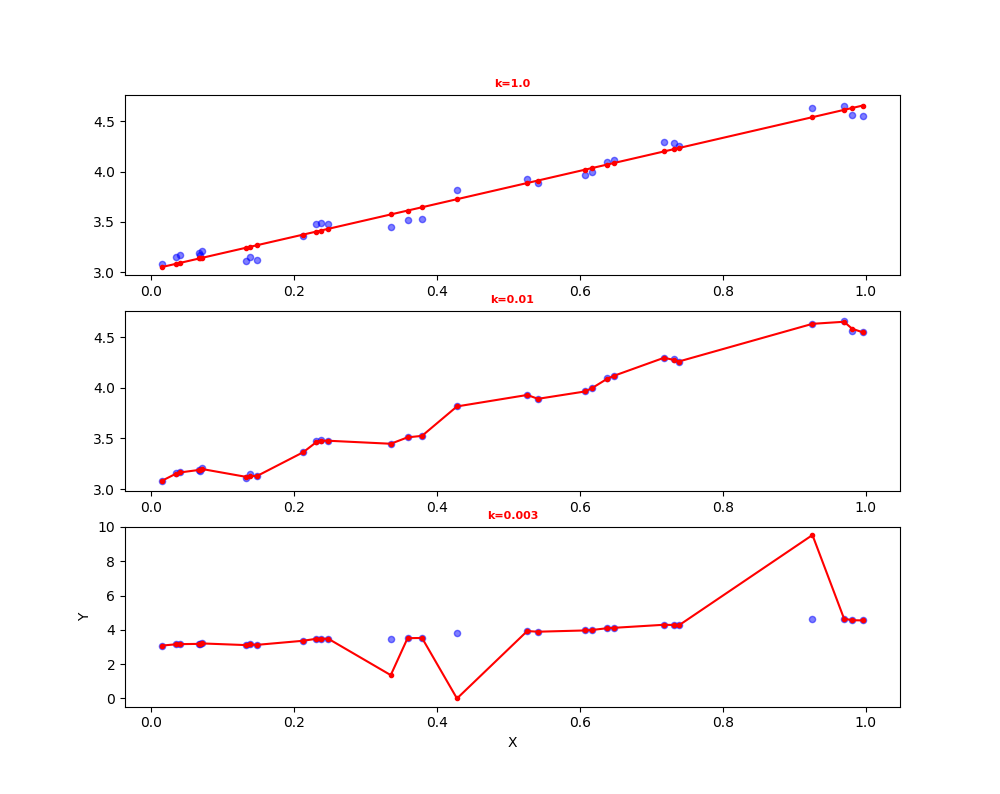# python机器学习案例系列教程——线性函数、线性回归、正则化

python数据挖掘系列教程

1、样本数据集，每列代表一个对象。也就是说一个待测对象是一个列向量
2、样本数据集，每行代表一个对象。也就是说待测对象是一个行向量

## 线性函数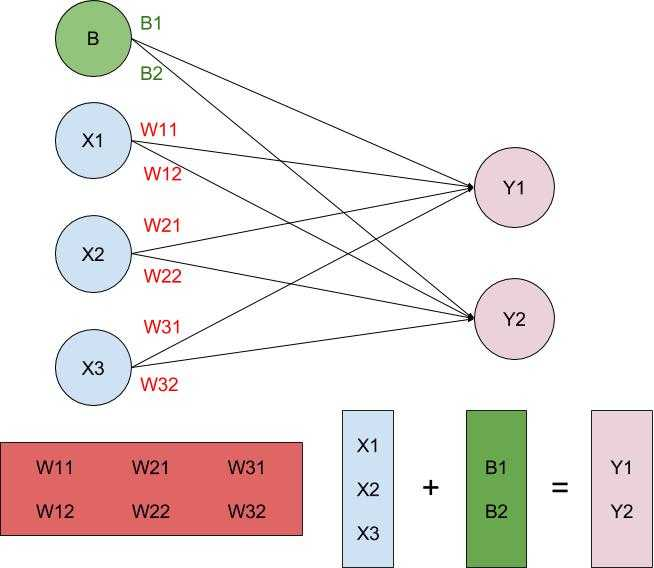1、在很多线性回归模型中根本看不到B的存在，而仅写成Y=WX，从上图中也可以看出，这是因为B可以看成是输入对象一个值为1的属性所对应的权重。即y1=W11*X1+W21*X2+W31*X3+1*B1，这里Y的每个分量由输入对象每个属性线性组合得出。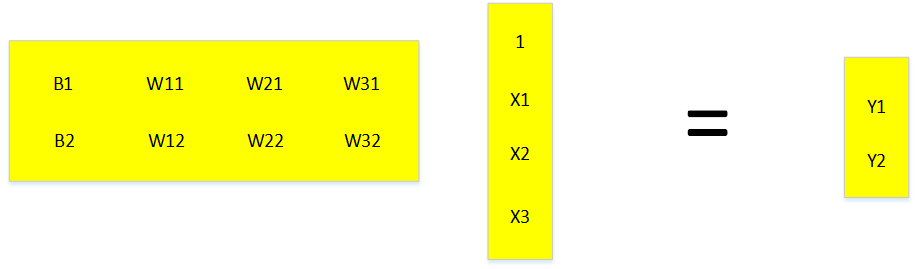2、我们可以这样理解线性函数，权重w的某一行表示输入对象每个属性对输出分量的贡献率。比如w11表示待测样本属性x1对分量y1的贡献率，w21表示待测样本属性x2对分量y1的贡献率，w12表示待测样本属性x1对分量y2的贡献率。如果我们不需要预测Y2分量，权重矩阵就不存在W12，W22，W32。我们所以如果我们想要预测的结果不需要那么多分量，只是减少了权重矩阵的维度。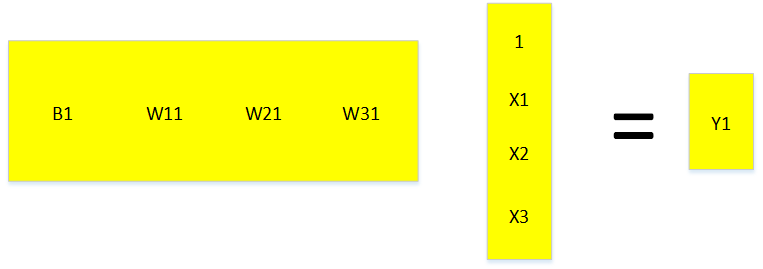3、对于线性二分类，就是找到一个直接或平面超平面将两个集合分割开。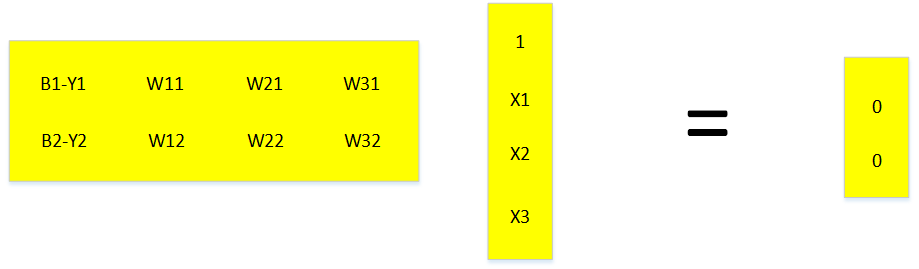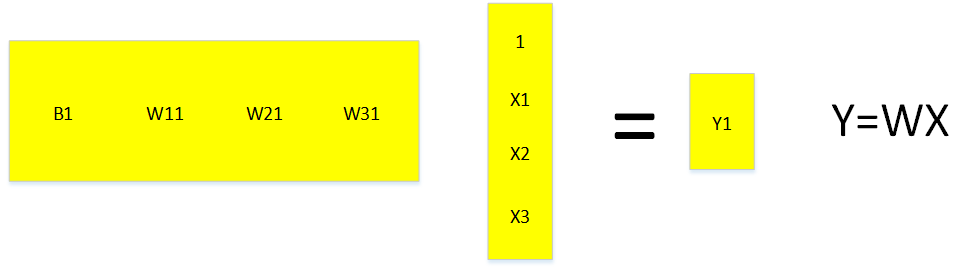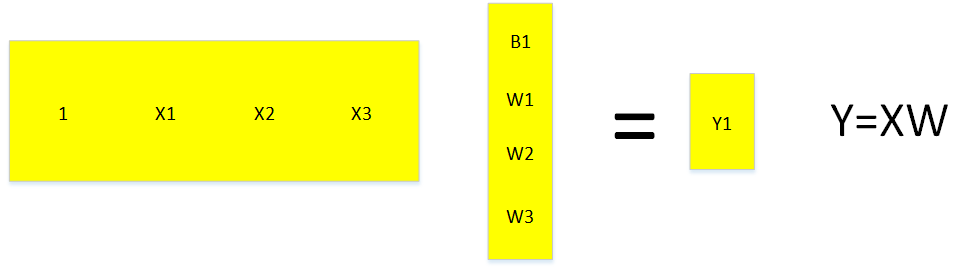## 线性回归

$y=b1\ast 1+w11\ast x1+w12\ast x2+w13\ast x3$

（若X每列为一个对象，w为行向量，则误差平方和为）

$\sum _{i=1}^{m}\left({y}_{i}-w{x}_{i}{\right)}^{2}$$\sum_{i=1}^{m}(y_i-wx_i)^2$

（若X每行为一个对象，w为列向量，则误差平方和为）

$\sum _{i=1}^{m}\left({y}_{i}-{x}_{i}w{\right)}^{2}$$\sum_{i=1}^{m}(y_i-x_iw)^2$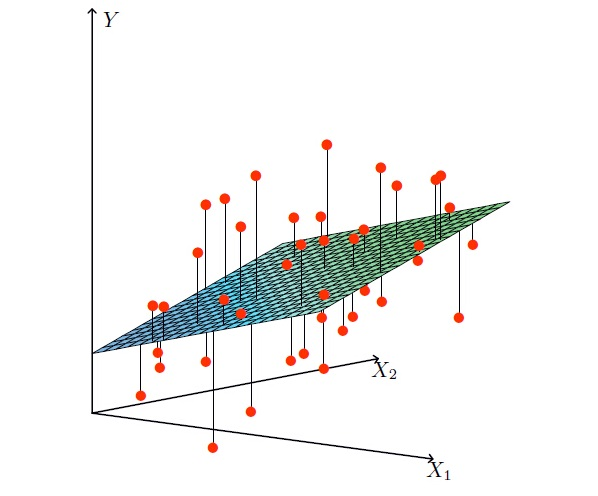## 线性回归中为什么一般选用误差平方和作为损失函数？

$p\left({y}^{\left(i\right)}|{x}^{\left(i\right)};\theta \right)=\frac{1}{\sqrt{2\pi }\sigma }exp\left(-\frac{\left({y}^{\left(i\right)}-{\theta }^{T}{x}^{\left(i\right)}{\right)}^{2}}{2{\sigma }^{2}}\right)$

## 除了误差平方和还有哪些损失函数

1） 0-1损失函数

$L(Y,f(X))= \begin{cases} 1, & \text {Y≠f(X)} \\ 0, & \text{ Y=f(X)} \end{cases}$

2） 平方损失函数

$L\left(Y,f\left(X\right)\right)=\left(Y-f\left(X\right){\right)}^{2}$$L(Y,f(X))=(Y−f(X))^2$

3） 绝对损失函数

$L\left(Y,f\left(X\right)\right)=|Y-f\left(X\right)|$$L(Y,f(X))=|Y−f(X)|$

4） 对数损失函数

$L\left(Y,P\left(Y|X\right)\right)=-logP\left(Y|X\right)$$L(Y,P(Y|X))=−logP(Y|X)$

## 最小二乘法

y=wx，其中w为横向量，x为列向量，y为数值。
y=xw，其中w为列向量，x为横向量，y为数值。

1、若用X表示多个样本对象，每行为一个对象。则要写成y=Xw，其中w为列向量，x为矩阵，y为列向量，每个分量表示每个对象的预测结果。此时误差平方和对权重矩阵w求导，为下面的公式。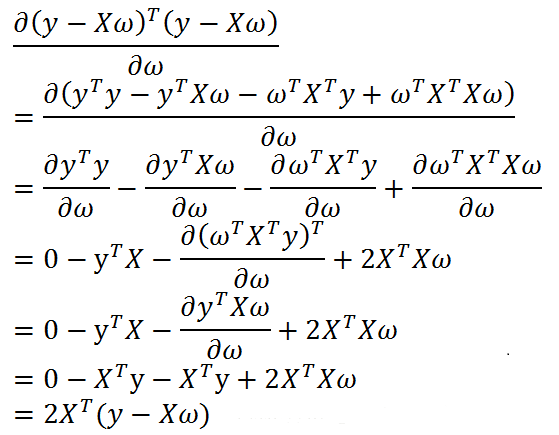$w=\left({X}^{T}X{\right)}^{-1}{X}^{T}y$$w=(X^TX)^{-1}X^Ty$

2、若用X表示多个样本对象，每列为一个对象。则y=wX中，w为横向量，x为矩阵，y为横向量，每个分量表示每个对象的预测结果。此时误差平方和对权重矩阵w求导，为下面的公式。

$\frac{d\left(y-wX{\right)}^{T}\left(y-wX\right)}{dw}$

$w={y}^{T}{X}^{T}\left(X{X}^{T}{\right)}^{-1}$$w=y^TX^T(XX^T)^{-1}$

## 梯度下降法

$J\left(w\right)=\sum _{i=1}^{m}\left({x}_{i}w-{y}_{i}{\right)}^{2}$$J(w)=\sum_{i=1}^m(x_iw-y_i)^2$

$\frac{dJ\left(w\right)}{dw}=2{X}^{T}\left(Xw-y\right)$$\frac{d J(w)}{d w} = 2X^T(Xw-y)$

$\mathrm{\nabla }J\left(w\right)=2{X}^{T}\left(Xw-y\right)$$\nabla J(w)=2X^T(Xw-y)$

${w}_{k+1}={w}_{k}-\rho \ast \mathrm{\nabla }J\left({w}_{k}\right)={w}_{k}-\rho \ast 2{X}^{T}\left(X{w}_{k}-y\right)$$w_{k+1}=w_k-\rho * \nabla J(w_k)=w_k-\rho * 2X^T(Xw_k-y)$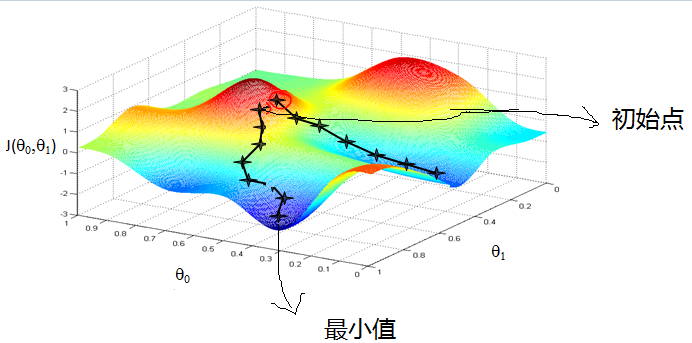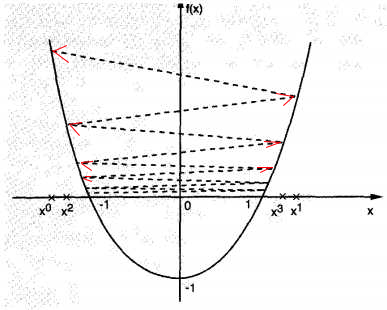## 随机梯度下降法

${w}_{k+1}={w}_{k}-2\ast {\rho }_{k}{x}_{k}^{T}\left({x}_{k}{w}_{k}-{y}_{k}\right)$$w_{k+1}=w_k-2*\rho_kx_k^T(x_kw_k-y_k)$

$\sum _{k=1}^{\mathrm{\infty }}{\rho }_{k}\to \mathrm{\infty }$$\displaystyle\sum_{k=1}^{\infty} \rho_k \to\infty$

$\sum _{k=1}^{\mathrm{\infty }}{\rho }_{k}^{2}<\mathrm{\infty }$$\displaystyle\sum_{k=1}^{\infty} \rho_k^2 < \infty$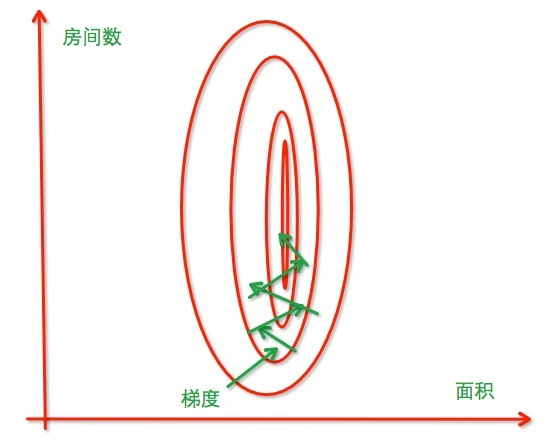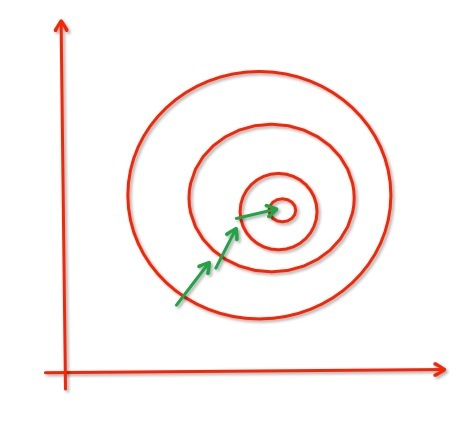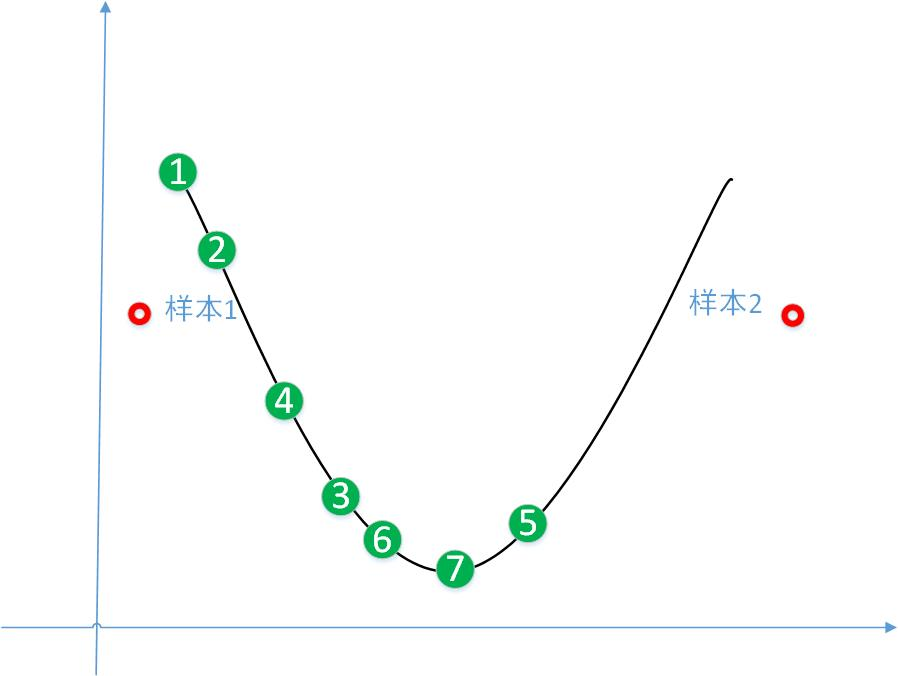## 线性回归案例

1. 当为array的时候，默认d*f就是对应元素的乘积，multiply也是对应元素的乘积，dot（d,f）会转化为矩阵的乘积， dot点乘意味着相加，而multiply只是对应元素相乘，不相加。

2. 当为mat的时候，默认d*f就是矩阵的乘积，multiply转化为对应元素的乘积，dot（d,f）为矩阵的乘积

## 一、单变量的线性回归

1、加载数据集

0.067732    3.176513
0.427810    3.816464
0.995731    4.550095
0.738336    4.256571
0.981083    4.560815
0.526171    3.929515
0.378887    3.526170
0.033859    3.156393
0.132791    3.110301
0.138306    3.149813
0.247809    3.476346
0.648270    4.119688
0.731209    4.282233
0.236833    3.486582
0.969788    4.655492
0.607492    3.965162
0.358622    3.514900
0.147846    3.125947
0.637820    4.094115
0.230372    3.476039
0.070237    3.210610
0.067154    3.190612
0.925577    4.631504
0.717733    4.295890
0.015371    3.085028
0.335070    3.448080
0.040486    3.167440
0.212575    3.364266
0.617218    3.993482
0.541196    3.891471

import matplotlib.pyplot as plt
import numpy as np

#加载数据集，最后一列最为目标值，前面的为特征属性的值
def loadDataSet(fileName):
xArr = []; yArr = []
for line in open(fileName).readlines():
curLine = line.strip().split('\t')
xonerow = [1.0]   #添加1.0作为第一个系数，则第一个系数的权重用来代表y=wx+b中的b变量
for i in range(len(curLine)-1):
xonerow.append(float(curLine[i]))  #最后一列为输出结果值y，前面的值为输入x值
xArr.append(xonerow)
yArr.append(float(curLine[-1]))  #添加最后一列为结果值

return xArr, yArr




#绘制二维数据集
def plotDataSet():
xArr, yArr = loadDataSet('data.txt')                                #加载数据集
xcord = [xArr[i] for i in range(len(xArr))]
ycord = [yArr[i] for i in range(len(yArr))]                         #样本点
fig = plt.figure()
ax = fig.add_subplot(111)                                            #添加subplot
ax.scatter(xcord, ycord, s = 20, c = 'blue',alpha = .5)                #绘制样本点
plt.xlabel('X');plt.ylabel('Y')
plt.show()

if __name__ == '__main__':
plotDataSet()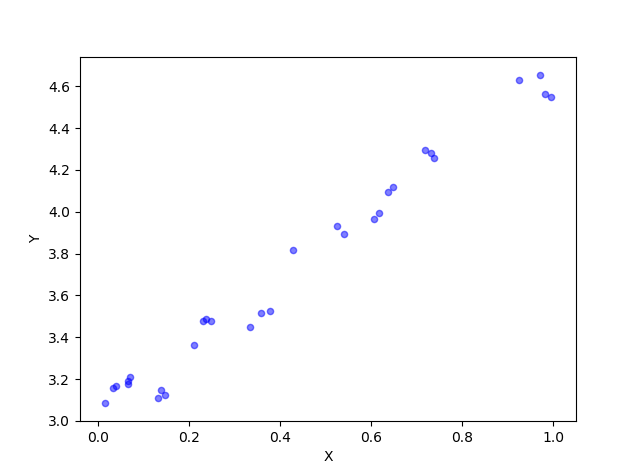2、获取线性回归模型

$w=\left({X}^{T}X{\right)}^{-1}{X}^{T}y$$w=(X^TX)^{-1}X^Ty$

standRegres函数根据输入输出，计算回归系数w。


#最小二乘法计算回归系数。xArr为样本数据集，包含m个对象，n种属性。yarr为结果数据集
def standRegres(xArr,yArr):
xMat = np.mat(xArr)       #转化为x矩阵。自动形成m行n列
yMat = np.mat(yArr).reshape(len(yArr),1)     #转化为y列向量
xTx = xMat.T * xMat       #根据文中推导的公示计算回归系数
if np.linalg.det(xTx) == 0.0:   #对不能求逆的结果返回
print("矩阵为奇异矩阵,不能求逆")
return
ws = xTx.I * (xMat.T*yMat)  #最小二乘求导出为0时的权重向量
return ws

plotRegression函数先求解了回归系数w，然后绘制样本数据集点，又绘制了w系数（所谓绘制w就是绘制w所代表的直线）

# 绘制样本数据集，求解回归曲线，绘制回归曲线。regression为计算回归系数的函数
def plotRegression(regression):
# 计算回归系数
xArr, yArr = loadDataSet('data.txt')                                    #加载数据集
ws = regression(xArr, yArr)                                           #计算回归系数列向量
print(ws)

xMat = np.mat(xArr)                                                    #创建xMat矩阵
yMat = np.mat(yArr)                                                    #创建yMat矩阵（行向量）

# 绘制样本数据集
xarr = xMat[:, 1].flatten().A  # 将矩阵第一列转化为一行矩阵，并获取第一行的列表
yarr = yMat.flatten().A  # 将矩阵第一列转化为一行矩阵，并获取第一行的行向量
plt.scatter(xarr, yarr, s=20, c='blue', alpha=.5)  # 绘制样本点

# 绘制回归系数。通过两个待测点，预测其值。以直线的形式反映出回归系数。
testArr = np.array([[1,0],[1,1]])                                        #将对象[1,0]和对象[1,1]最为待测对象，第一个1为常值代表偏量，后面的分量代表属性x的值
yHat = np.dot(testArr,ws)                                                      #计算使用线性模型预测的y值。dot就是矩阵乘法。
plt.plot(testArr[:,1], yHat, c = 'red')                                #绘制回归曲线，x为第1列，y为结果列向量，
plt.xlabel('X');plt.ylabel('Y')
plt.show()

if __name__ == '__main__':
plotRegression(standRegres)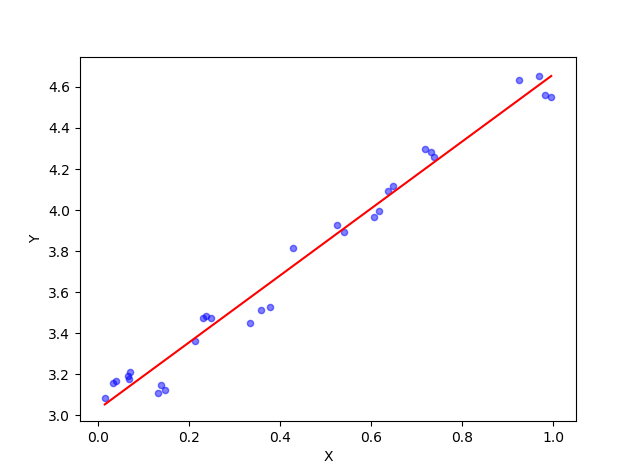[[ 3.02863046]
[ 1.6314263 ]]

$\mathrm{\nabla }J\left(w\right)=2{X}^{T}\left(Xw-y\right)$$\nabla J(w)=2X^T(Xw-y)$

${w}_{k+1}={w}_{k}-\rho \mathrm{\nabla }J\left({w}_{k}\right)$$w_{k+1}=w_k-\rho\nabla J(w_k)$


#梯度下降法计算回归系数。xArr为属性数据集，每行为一个对象。yArr为结果数据集，每行为一个对象的结果。
def gradAscent(xArr,yArr):
xMatrix = np.mat(xArr)                                        #转换成numpy的矩阵。xMatrix每行为一个对象，每列为一种特征属性
yMatrix = np.mat(yArr).reshape(len(yArr),1)            #转换成numpy的矩阵,并变维成列向量
m, n = np.shape(xMatrix)                                            #返回dataMatrix的大小。m为样本对象的个数,n为列数。
alpha = 0.001                                                        #移动步长,也就是学习速率,控制更新的幅度。
maxCycles = 500                                                      #最大迭代次数
weights = np.ones((n,1))                                             #初始化权重列向量
for k in range(maxCycles):
h =  xMatrix * weights                               #梯度上升矢量化公式，计算预测值（列向量）
error = h - yMatrix                                            #计算误差
weights = weights - alpha * 2 * xMatrix.T * error                 # 调整回归系数
return weights.getA()                                                #将矩阵转换为数组，返回权重数组


if __name__ == '__main__':
plotRegression(gradAscent)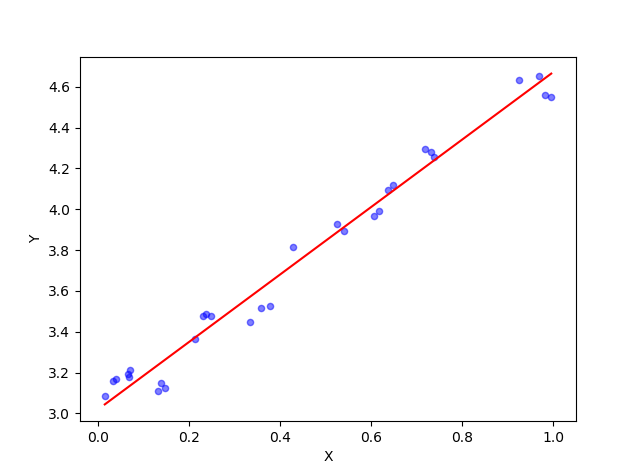[[ 3.01830318]
[ 1.65370732]]

${w}_{k+1}={w}_{k}-2\ast {\rho }_{k}{x}_{k}^{T}\left({x}_{k}{w}_{k}-{y}_{k}\right)$$w_{k+1}=w_k-2*\rho_kx_k^T(x_kw_k-y_k)$

（其中${x}_{k}$$x_k$为第k个样本对象，为行向量。${y}_{k}$$y_k$为该对象产生的预测输出数值。${w}_{k}$$w_k$为迭代第k次产生的权重列向量w，当对新的一行对象进行预测时，总是使用最新的w。）

$\sum _{k=1}^{\mathrm{\infty }}{\rho }_{k}\to \mathrm{\infty }$$\displaystyle\sum_{k=1}^{\infty} \rho_k \to\infty$

$\sum _{k=1}^{\mathrm{\infty }}{\rho }_{k}^{2}<\mathrm{\infty }$$\displaystyle\sum_{k=1}^{\infty} \rho_k^2 < \infty$

${\rho }_{k}=\frac{4}{\left(1.0+i+k\right)}+0.01$$\rho_k =\frac{4}{(1.0 + i + k)} + 0.01$

#随机梯度下降法计算回归系数
def randgradAscent(xArr,yArr):
xMatrix = np.mat(xArr)                                        #转换成numpy的矩阵。xMatrix每行为一个对象，每列为一种特征属性
yMatrix = np.mat(yArr).reshape(len(yArr),1)                   #转换成numpy的矩阵,并变维成列向量
m, n = np.shape(xMatrix)                                            #返回dataMatrix的大小。m为样本对象的个数,n为列数。
maxCycles = 100                                                      #最大迭代次数
weights = np.ones((n,1))                                             #初始化权重列向量
for i in range(maxCycles):
for k in range(m):
alpha = 4 / (1.0 + i + k) + 0.01                       # 降低alpha的大小，每次减小1/(j+i)。刚开始的时候可以步长大一点，后面调整越精细
h =  xMatrix[k] * weights                                      #随机梯度上升矢量化公式，计算预测值y
error = h - yMatrix[k]                                            #计算误差
weights = weights - 2*alpha * xMatrix[k].T * error                 # 调整回归系数
return weights.getA()                                                #将矩阵转换为数组，返回权重数组

if __name__ == '__main__':
plotRegression(randgradAscent)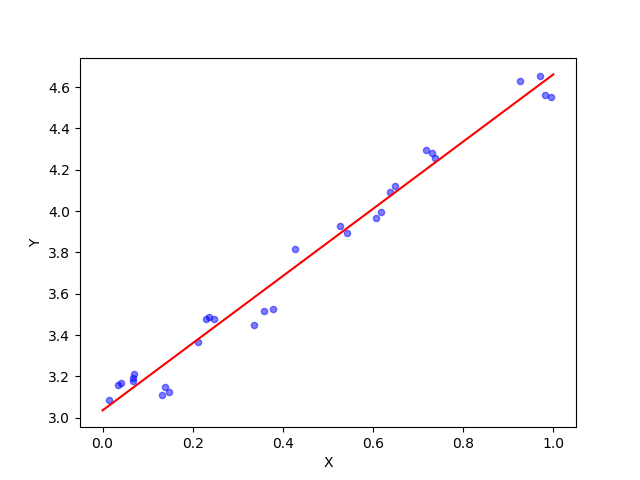[[ 3.03501804]
[ 1.62654039]]

## 拟合：拟合模型/函数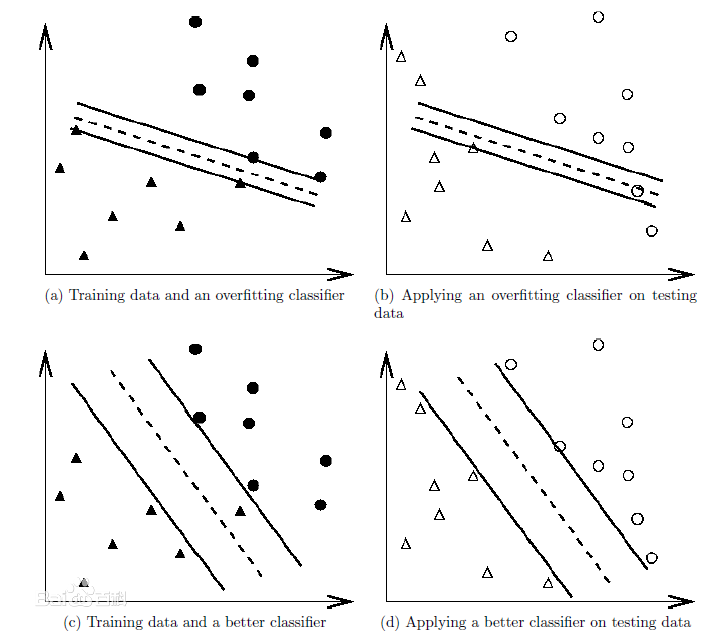1、合适拟合
2、欠拟合，或者叫作叫做高偏差(bias)。
3、过拟合，也叫高方差(variance)。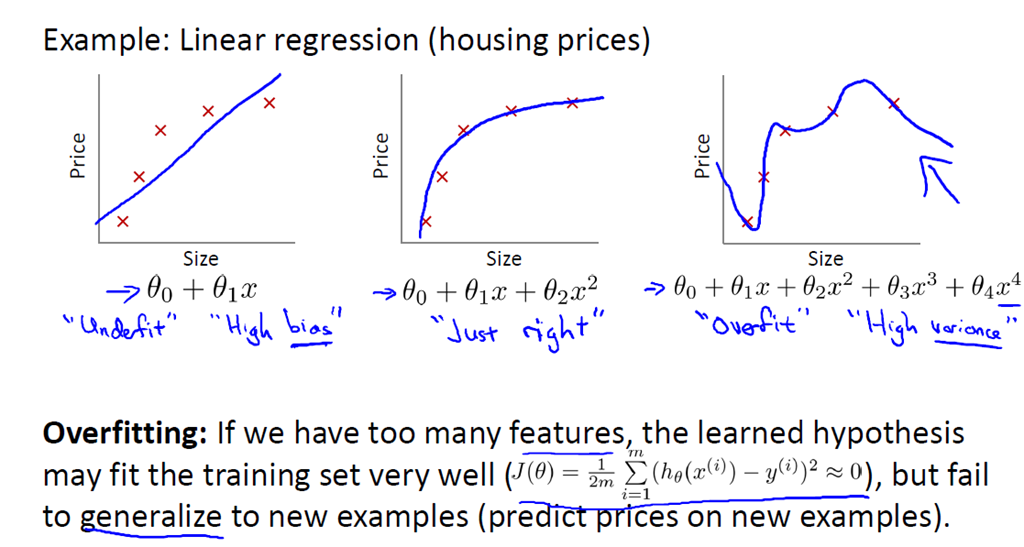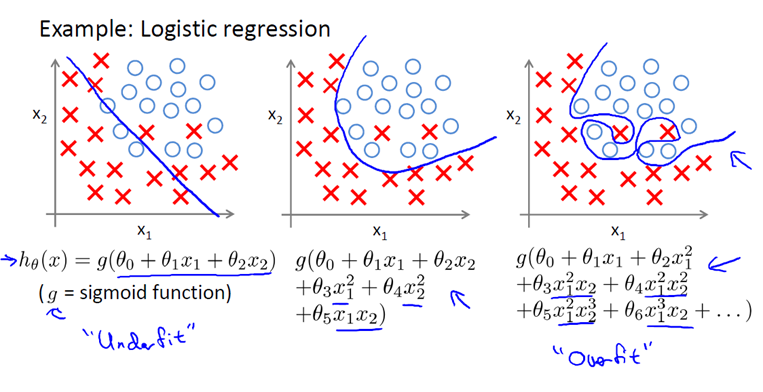## 正则化（处理过拟合）：

1） 减少特征的数量，有人工选择，或者采用模型选择算法
http://www.cnblogs.com/heaad/archive/2011/01/02/1924088.html （特征选择算法的综述）

2） 正则化，即保留所有特征，但降低参数的值的影响。正则化的优点是，特征很多时，每个特征都会有一个合适的影响因子。

$J\left(w\right)=\frac{1}{2m}\left[\sum _{i=1}^{m}\left({h}_{w}\left({x}^{\left(i\right)}\right)-{y}^{\left(i\right)}{\right)}^{2}\right]+\lambda \sum _{j=1}^{n}{w}_{j}^{2}$

$\lambda$$\lambda$就是惩罚因子。

$w=\left({X}^{T}X+\lambda \left(\begin{array}{c}0\\ & 1& \\ & & 1& \\ & & & \cdots \\ & & & & 1\end{array}\right){\right)}^{-1}{X}^{T}y$

$J\left(w\right)=-\left[\frac{1}{m}\sum _{i=1}^{m}{y}^{\left(i\right)}log{h}_{w}\left({x}^{\left(i\right)}\right)+\left(1-{y}^{\left(i\right)}\right)log\left(1-{h}_{w}\left({x}^{\left(i\right)}\right)\right)\right]+\frac{\lambda }{2m}\sum _{j=1}^{n}{w}_{j}^{2}$

L1正则化、L2正则化

L1正则化和L2正则化可以看做是损失函数的惩罚项。对于线性回归模型，使用L1正则化的模型建叫做Lasso回归，使用L2正则化的模型叫做Ridge回归（岭回归）。

L1正则化是指权值向量w中各个元素的绝对值之和，通常表示为$||w|{|}_{1}$$||w||_1$

$J\left(w\right)=\frac{1}{2m}\left[\sum _{i=1}^{m}\left({h}_{w}\left({x}^{\left(i\right)}\right)-{y}^{\left(i\right)}{\right)}^{2}+\lambda \sum _{j=1}^{n}|{w}_{j}|\right]$

L2正则化是指权值向量w中各个元素的平方和然后再求平方根（可以看到Ridge回归的L2正则化项有平方符号），通常表示为$||w|{|}_{2}$$||w||_2$

$J\left(w\right)=\frac{1}{2m}\left[\sum _{i=1}^{m}\left({h}_{w}\left({x}^{\left(i\right)}\right)-{y}^{\left(i\right)}{\right)}^{2}\right]+\lambda \sum _{j=1}^{n}{w}_{j}^{2}$

L1正则化可以产生稀疏权值矩阵，即产生一个稀疏模型，可以用于特征选择。这是因为w绝对值求和这个正则项求导产生的后果是：当w为正时，更新后的w变小。当w为负时，更新后的w变大。因此它的效果就是让w往0靠，使网络中的权重尽可能为0，也就相当于减小了网络复杂度，防止过拟合。

L2正则化可以防止模型过拟合（overfitting）；一定程度上，L1也可以防止过拟合

L2 和 L1 采用不同的方式降低权重：

• L2 会降低权重2。
• L1 会降低 |权重|。

• L2 的导数为 2 * 权重。
• L1 的导数为 k（一个常数，其值与权重无关）。

L1 正则化 - 减少所有权重的绝对值 - 证明对宽度模型非常有效。

## 坐标轴下降法（解决L1正则化不可导的问题）

$J\left(w\right)=\frac{1}{2m}\left[\sum _{i=1}^{m}\left({h}_{w}\left({x}^{\left(i\right)}\right)-{y}^{\left(i\right)}{\right)}^{2}+\lambda \sum _{j=1}^{n}|{w}_{j}|\right]$

## 局部加权线性回归（处理欠拟合）

$w=\left({X}^{T}WX{\right)}^{-1}{X}^{T}Wy$

LWLR使用”核”（与支持向量机中的核类似）来对附近的点赋予更高的权重。核的类型可以自由选择，最常用的核就是高斯核，高斯核对应的权重如下：其中$W\left(i,i\right)$$W(i,i)$表示权重矩阵第i个对角值，${x}_{i}$$x_i$表示第i个样本对象，$x$$x$表示待测对象。

$W\left(i,i\right)=exp\left(-\frac{\left({x}_{i}-x{\right)}^{2}}{2{k}^{2}}\right)$

import matplotlib.pyplot as plt
import numpy as np

#加载数据集，最后一列最为目标值，前面的为特征属性的值
def loadDataSet(fileName):
xArr = []; yArr = []
for line in open(fileName).readlines():
curLine = line.strip().split('\t')
xonerow = [1.0]   #添加1.0作为第一个系数，则第一个系数的权重用来代表y=wx+b中的b变量
for i in range(len(curLine)-1):
xonerow.append(float(curLine[i]))  #最后一列为输出结果值y，前面的值为输入x值
xArr.append(xonerow)
yArr.append(float(curLine[-1]))  #添加最后一列为结果值

return xArr, yArr

# 局部加权线性回归。绘制多条局部加权回归曲线
def plotlwlrRegression():
xArr, yArr = loadDataSet('data.txt')                                    #加载数据集
xMat = np.mat(xArr)                                                    #创建xMat矩阵。属性数据集，每行为一个样本对象，每列为一种属性
yMat = np.mat(yArr).reshape(len(yArr),1)                               #创建yMat矩阵。将结果值存储成列向量

testMat = xMat                     #原样选取样本数据集作为测试集。看看预测结果与真实结果之间的差距
m = np.shape(testMat)          # 计算待测数据集的样本个数
yHat_1 = []  # 定义一个列表用来存储预测值
yHat_2 = []  # 定义一个列表用来存储预测值
yHat_3 = []  # 定义一个列表用来存储预测值
for i in range(m):  # 对每个待测样本点进行预测
yHat_1.append(lwlr(testMat[i,:], xMat, yMat, 1.0))                    # 根据局部加权线性回归计算yHat
yHat_2.append(lwlr(testMat[i,:], xMat, yMat, 0.01))                   # 根据局部加权线性回归计算yHat
yHat_3.append(lwlr(testMat[i,:], xMat, yMat, 0.003))                   # 根据局部加权线性回归计算yHat
yHat_1 = np.mat(yHat_1).reshape(len(yHat_1),1)
yHat_2 = np.mat(yHat_2).reshape(len(yHat_2), 1)
yHat_3 = np.mat(yHat_3).reshape(len(yHat_3), 1)

srtInd = xMat[:, 1].argsort(0)                                              #将样本数据集，第2列排序，返回索引值
xSort = xMat[srtInd][:,0,:]                                                 #根据排序索引，将所有列排序
ySort_1 = yHat_1[srtInd][:,0,:]
ySort_2 = yHat_2[srtInd][:,0,:]
ySort_3 = yHat_3[srtInd][:,0,:]

fig, axs = plt.subplots(nrows=3, ncols=1,figsize=(10,8))                    #创建三个子图
axs.plot(xSort[:,1], ySort_1, c = 'red',marker='.')                        #绘制回归曲线
axs.plot(xSort[:,1], ySort_2, c = 'red',marker='.')                        #绘制回归曲线
axs.plot(xSort[:,1], ySort_3, c = 'red',marker='.')                        #绘制回归曲线

axs.scatter(xMat[:,1].flatten().A, yMat.flatten().A, s = 20, c = 'blue', alpha = .5)                #绘制样本点
axs.scatter(xMat[:,1].flatten().A, yMat.flatten().A, s = 20, c = 'blue', alpha = .5)                #绘制样本点
axs.scatter(xMat[:,1].flatten().A, yMat.flatten().A, s = 20, c = 'blue', alpha = .5)                #绘制样本点

#设置标题,x轴label,y轴label
axs0_title_text = axs.set_title(u'k=1.0', size=8, weight='bold', color='red')
axs1_title_text = axs.set_title(u'k=0.01', size=8, weight='bold', color='red')
axs2_title_text = axs.set_title(u'k=0.003', size=8, weight='bold', color='red')

plt.xlabel('X');plt.ylabel('Y')
plt.show()

# 使用局部加权线性回归计算回归系数w。不同的待测点获得的回归系数都不同。testPoint待测对象，xArr样本数据集，yArr结果数据集，k - 高斯核的k，表示拟合程度，为0表示100%拟合,自定义参数
def lwlr(testPoint, xMat, yMat, k = 1.0):
m = np.shape(xMat)                                               # 获取样本数量
weights = np.mat(np.eye((m)))                                       # 初始化局部权重对角矩阵。这里的局部权重矩阵是为待测点周边的每个节点赋予的影响矩阵。并不是线性回归系数。
for j in range(m):                                                 # 遍历数据集计算每个样本的权重
diffMat = testPoint - xMat[j,:]                                # 计算样本中每个对象与待测对象之间的距离
weights[j, j] = np.exp(diffMat * diffMat.T/(-2.0 * k**2))       # 为每个周边节点设定权重。越近的节点权重越大，越远的节点权重越小
xTx = xMat.T * (weights * xMat)                                     #  增加了局部权重矩阵，增大临近节点的影响力，降低远方节点的影响力
if np.linalg.det(xTx) == 0.0:
print("矩阵为奇异矩阵,不能求逆")
return
ws = xTx.I * (xMat.T * (weights * yMat))                            # 计算回归系数
y = testPoint * ws                                                  # 计算预测值，矩阵相乘得到的是矩阵。虽然只有一个元素
return y[0,0]       #读取矩阵的值，返回数值

if __name__ == '__main__':
plotlwlrRegression()